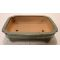##### The Japanese Bonsai specialist
Direct order Contact Help / Services Newsletter# Small green bonsai pot

› Bonsai pots › Rectangular potsref. : 7628

10,00

Available quantity : 2Order

###### Description

Exterior: 21*16*6 cm. Interior: 18*13*4.5 cm. A green glazed bonsai pot in a classic rectangular form. Buy the matching drip tray Ref 6973 or Ref 8594

#rectangular 4.1 #bonsai 3.6 #green 3.5 #small 2.5 #pots 2.4 #exterior 1.8 #interior 1.8 #matching 1.8 #classic 1.7 #glazed 1.6

###### Technical description
 shape rectangular color green origin China Yixing

Formule
(( ROUND((CHAR_LENGTH(b.article_nom)-CHAR_LENGTH(REPLACE(b.article_nom, 'bonsai', '')))/LENGTH('bonsai')) + ROUND((CHAR_LENGTH(b.article_description)-CHAR_LENGTH(REPLACE(b.article_description, 'bonsai', '')))/LENGTH('bonsai')) ) * 3.6) + (( ROUND((CHAR_LENGTH(b.article_nom)-CHAR_LENGTH(REPLACE(b.article_nom, 'green', '')))/LENGTH('green')) + ROUND((CHAR_LENGTH(b.article_description)-CHAR_LENGTH(REPLACE(b.article_description, 'green', '')))/LENGTH('green')) ) * 3.5) + (( ROUND((CHAR_LENGTH(b.article_nom)-CHAR_LENGTH(REPLACE(b.article_nom, 'small', '')))/LENGTH('small')) + ROUND((CHAR_LENGTH(b.article_description)-CHAR_LENGTH(REPLACE(b.article_description, 'small', '')))/LENGTH('small')) ) * 2.5) + (( ROUND((CHAR_LENGTH(b.article_nom)-CHAR_LENGTH(REPLACE(b.article_nom, 'rectangular', '')))/LENGTH('rectangular')) + ROUND((CHAR_LENGTH(b.article_description)-CHAR_LENGTH(REPLACE(b.article_description, 'rectangular', '')))/LENGTH('rectangular')) ) * 2.1) + (( ROUND((CHAR_LENGTH(b.article_nom)-CHAR_LENGTH(REPLACE(b.article_nom, 'exterior', '')))/LENGTH('exterior')) + ROUND((CHAR_LENGTH(b.article_description)-CHAR_LENGTH(REPLACE(b.article_description, 'exterior', '')))/LENGTH('exterior')) ) * 1.8) + (( ROUND((CHAR_LENGTH(b.article_nom)-CHAR_LENGTH(REPLACE(b.article_nom, 'interior', '')))/LENGTH('interior')) + ROUND((CHAR_LENGTH(b.article_description)-CHAR_LENGTH(REPLACE(b.article_description, 'interior', '')))/LENGTH('interior')) ) * 1.8) + (( ROUND((CHAR_LENGTH(b.article_nom)-CHAR_LENGTH(REPLACE(b.article_nom, 'matching', '')))/LENGTH('matching')) + ROUND((CHAR_LENGTH(b.article_description)-CHAR_LENGTH(REPLACE(b.article_description, 'matching', '')))/LENGTH('matching')) ) * 1.8) + (( ROUND((CHAR_LENGTH(b.article_nom)-CHAR_LENGTH(REPLACE(b.article_nom, 'classic', '')))/LENGTH('classic')) + ROUND((CHAR_LENGTH(b.article_description)-CHAR_LENGTH(REPLACE(b.article_description, 'classic', '')))/LENGTH('classic')) ) * 1.7) + (( ROUND((CHAR_LENGTH(b.article_nom)-CHAR_LENGTH(REPLACE(b.article_nom, 'glazed', '')))/LENGTH('glazed')) + ROUND((CHAR_LENGTH(b.article_description)-CHAR_LENGTH(REPLACE(b.article_description, 'glazed', '')))/LENGTH('glazed')) ) * 1.6) + (( ROUND((CHAR_LENGTH(b.article_nom)-CHAR_LENGTH(REPLACE(b.article_nom, 'tray ', '')))/LENGTH('tray ')) + ROUND((CHAR_LENGTH(b.article_description)-CHAR_LENGTH(REPLACE(b.article_description, 'tray ', '')))/LENGTH('tray ')) ) * 1.6)

## Secure payment## Delivery

Our logistic partners :04 74 55 23 48
Pépinière MAILLOT-BONSAÏ
Le Bois Frazy
01990 RELEVANT - FRANCE
on appointment